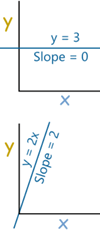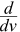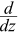# Derivative Rules

(toc) #title=(Table of Content)

The Derivative tells us the slope of a function at any point.There are rules we can follow to find many derivatives. For example:
• The slope of a constant value (like 3) is always 0
• The slope of a line like 2x is 2, or 3x is 3 etc
• and so on.
Here are useful rules to help you work out the derivatives of many functions (with examples below). Note: the little mark  means "Derivative of".
Common FunctionsFunctionDerivative
Constantc0
Linex1
axa
Squarex22x
Square Root√x(½)x
Exponentialexex
axln(a) ax
Logarithmsln(x)1/x
loga(x)1 / (x ln(a))
cos(x)−sin(x)
tan(x)sec2(x)
Inverse Trigonometrysin-1(x)1/√(1−x2)
cos-1(x)−1/√(1−x2)
tan-1(x)1/(1+x2)
RulesFunctionDerivative
Multiplication by constantcfcf’
Power Rulexnnxn−1
Sum Rulef + gf’ + g’
Difference Rulef - gf’ − g’
Product Rulefgf g’ + f’ g
Quotient Rulef/g(f’ g − g’ f )/g2
Reciprocal Rule1/f−f’/f2
Chain Rule
(as "Composition of Functions")
f º g(f’ º g) × g’
Chain Rule (using ’ )f(g(x))f’(g(x))g’(x)
Chain Rule (using ddx )dydx = dydududx
"The derivative of" is also written ddx
So ddxsin(x) and sin(x)’ are the same thing, just written differently

## Examples

### Example: what is the derivative of sin(x) ?

From the table above it is listed as being cos(x)
It can be written as:sin(x) = cos(x)
Or:
sin(x)’ = cos(x)

### Example: What isx3 ?

The question is asking "what is the derivative of x3?"
We can use the Power Rule, where n=3:xn = nxn−1x3 = 3x3−1 = 3x2

### Example: What is(1/x) ?

1/x is also x-1
We can use the Power Rule, where n = −1:xn = nxn−1x−1 = −1x−1−1 = −x−2

### Example: What is5x3 ?

the derivative of cf = cf’
the derivative of 5f = 5f’
We know (from the Power Rule):x3 = 3x3−1 = 3x2
So:5x3 = 5x3 = 5 × 3x2 = 15x2

### Example: What is the derivative of x2+x3 ?

The Sum Rule says:
the derivative of f + g = f’ + g’
So we can work out each derivative separately and then add them.
Using the Power Rule:
•x2 = 2x
•x3 = 3x2
And so:
the derivative of x2 + x3 = 2x + 3x2

### Difference Rule

It doesn't have to be x, we can differentiate with respect to, for example, v:

### Example: What is(v3−v4) ?

The Difference Rule says
the derivative of f − g = f’ − g’
So we can work out each derivative separately and then subtract them.
Using the Power Rule:
•v3 = 3v2
•v4 = 4v3
And so:
the derivative of v3 − v4 = 3v2 − 4v3

### Example: What is(5z2 + z3 − 7z4) ?

Using the Power Rule:
•z2 = 2z
•z3 = 3z2
•z4 = 4z3
And so:(5z2 + z3 − 7z4) = 5 × 2z + 3z2 − 7 × 4z3 = 10z + 3z2 − 28z3

### Example: What is the derivative of cos(x)sin(x) ?

The Product Rule says:
the derivative of fg = f g’ + f’ g
In our case:
• f = cos
• g = sin
We know (from the table above):
•cos(x) = −sin(x)
•sin(x) = cos(x)
So:
the derivative of cos(x)sin(x) = cos(x)cos(x) − sin(x)sin(x)

cos2(x) − sin2(x)

### Example: What is(1/x) ?

The Reciprocal Rule says:
the derivative of 1/f = −f’/f2
With f(x)= x, we know that f’(x) = 1
So:
the derivative of 1/x = −1/x2
Which is the same result we got above using the Power Rule.

### Example: What is ddxsin(x2) ?

sin(x2) is made up of sin() and x2:
• f(g) = sin(g)
• g(x) = x2
The Chain Rule says:
the derivative of f(g(x)) = f'(g(x))g'(x)
The individual derivatives are:
• f'(g) = cos(g)
• g'(x) = 2x
So:
ddxsin(x2) = cos(g(x)) (2x)
= 2x cos(x2)
Another way of writing the Chain Rule is: dydx = dydududx
Let's do the previous example again using that formula:

### Example: What is ddxsin(x2) ?

dydx = dydududx
Have u = x2, so y = sin(u):
ddx sin(x2) = ddusin(u)ddxx2
Differentiate each:
ddx sin(x2) = cos(u) (2x)
Substitue back u = x2 and simplify:
ddx sin(x2) = 2x cos(x2)
Same result as before (thank goodness!)
Another couple of examples of the Chain Rule:

### Example: What is(1/cos(x)) ?

1/cos(x) is made up of 1/g and cos():
• f(g) = 1/g
• g(x) = cos(x)
The Chain Rule says:
the derivative of f(g(x)) = f’(g(x))g’(x)
The individual derivatives are:
• f'(g) = −1/(g2)
• g'(x) = −sin(x)
So:
(1/cos(x))’ = −1/(g(x))2 × −sin(x)
= sin(x)/cos2(x)
Note: sin(x)/cos2(x) is also tan(x)/cos(x), or many other forms.

### Example: What is(5x−2)3 ?

The Chain Rule says:
the derivative of f(g(x)) = f’(g(x))g’(x)
(5x-2)3 is made up of g3 and 5x-2:
• f(g) = g3
• g(x) = 5x−2
The individual derivatives are:
• f'(g) = 3g2 (by the Power Rule)
• g'(x) = 5
So:(5x−2)3 = 3g(x)2 × 5 = 15(5x−2)2
Tags